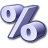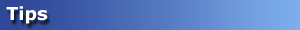# RatiosAllows the user to specify the value data shown in the grid:

• Margin %
Margin divided by Revenue x 100.
• Cost %
Cost of Goods Sold divided by Revenue x 100.
• Revenue per Unit
Total Revenue divided by Units.
• Margin per Unit
Total Margin divided by Units.
• Revenue Percentage of Total
Revenue value as a percentage of Column
• Margin Percentage of Total
Margin value as a percentage of Column
• Units Percentage of Total
Units as a percentage of ColumnIf, for any of these calculations, the denominator (either Revenue or Units) in the cell is zero, Salesmatrix will display zero, even though there may be a positive value in the numerator.

For all ratios that are "Percentage of total" the traffic lights are calculated across the rows, instead of down the columns.